While basic computer skills have long since been a pre-requisite for many jobs, learning a few key tricks in each of the programs that you use on a daily basis can really help you to transform your reputation around the office from Office Somebody to Office Hero. Enter Office Ninja Training. This week, let’s take a look at one of the most basic, yet critical functions in any spreadsheet application: the IF function.

Purpose: The IF function is meant to help make decisions within your spreadsheet. In other words, we can use this function to look at one piece of data in a specific cell, or combination of cells, and yield a result in a different cell that is based upon our given condition. For example, we might want to have our function look at a list of names and return if each person is male or female in the next column. Similarly, we might want our function to evaluate a series of cost expenditures in a budget and let us know if we’re over-budget or under-budget on each line item. Proper use of this function allows you to dictate what happens when a particular criteria that you create is either met, or not met.

How to use it: Before walking through an example, let’s take a look how to properly write an IF function.

=IF(condition to test, show if condition is true, show if condition is false)

The chunks of the function found between the commas, also known as “arguments” or “parameters”, may be described slightly differently between Microsoft Excel, Google Docs, and other spreadsheet editors, however their purpose is generally the same. Let’s break it down step by step using the following example.

Let’s look at the list of names below. We’ve kept this example simple by only using two different names in our list in Column A. Let’s evaluate each name and determine the appropriate gender for both John and Mary.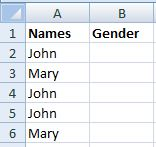Condition to test: In this argument, we are going to write our test criteria. This criteria must be something that evaluates to be either true or false. As such, when we enter our IF function into cell B2, we might enter the condition A2=”John”. If we were to read this criteria in plain English, we’d essentially be asking “Does cell A2 say John?” (We could have just as easily entered A2=”Mary” without any problems, but it would have some impact on how we write the subsequent arguments in this formula). In the case of A2=”John”, this would evaluate as True, but as we fill down to B3, the condition would evaluate to False as A3 clearly does not equal John. Also, notice that our condition is actually comparing two values. This enables the function to compare the two pieces of information and determine if the condition is true or false. If we just had one value, like a sum formula, we would encounter an error.

Show if condition is true: This argument will determine what value will be returned if our test condition evaluates as true. Continuing with our previous example in which our test condition is A2=”John”, we’d want our true condition to be “Male”. This means that whenever A2=”John”, cell B2 will say Male. If we put these two pieces of the function together and translate them into plain English, we’d be asking, “Does cell A2 say John? If so, write “Male” in cell B2.”

Show if condition is false: Finally, we have to tell our function what to do if our test condition is false. For example, if our test condition in cell B3 is A3=”John”, we’d want our false condition to be “Female” so that cell B3 ends up saying Female.

You’re doing great so far, and we’re almost done. But before we end this week’s tip, let’s put everything together and see out it looks. Here’s the formula that you’d enter into cell B2:

=If(A2="John","Male","Female")

The full formula, translated into plain English would read something like: “Does cell A2 say John? If so, write “Male” in cell B2. If not, write “Female” in cell B2.”

Finally, fill this formula down to cells B3 through B6 and your spreadsheet should end up looking like this: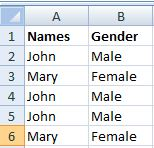And for your reference, here’s a screenshot of what each of the formulas should end up looking like after you fill them down: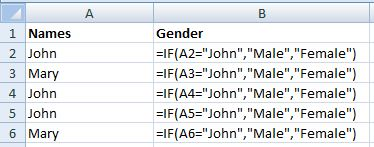Let’s try one more example. This time, let’s look at a monthly household budget. For each expense, we can see the monthly expenditure and budget. Let’s write an IF function that indicates how much money we still have available to spend for the month for a particular category, or if we are already over budget.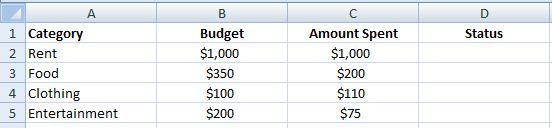In this example our condition we need to ask ourselves if we’ve spent more than our budget allows. As such, we’ll use this idea to drive our test condition. If we have spent too much, we want to show the message “Over Budget”. If we haven’t spent more than the budget, then let’s find out how much money we have remaining. First, I find it helpful to create an IF function in plain English:

“Have I spent more than the budget allows? If so, write “Over Budget”, but if not tell me the difference between the amount I’ve spent and my budget.”

From here, it’s only one more baby step to get to the follow IF formula for cell D2:

=IF(C2>B2,"Over Budget",B2-C2)

After filling this function down to cells D3 through D5, our worksheet should look like this: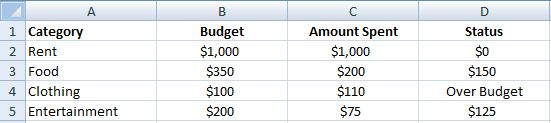And once again, in case you need the formulas as a reference: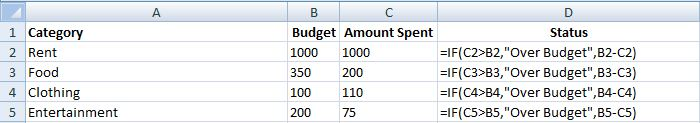Congratulations! You’ve now mastered the basics of the eternally useful IF function. You’d be surprised just how handy this little sucker can be when working in your spreadsheets, especially as you start writing more and more complex IF functions. Don’t worry though, we’ll be there to hold your hand every step of the way. Stay tuned for our upcoming article on wrapping or nesting IF functions within one another to create super IF fuctions.